# RD Sharma Solutions Class 9 Maths Chapter 3 Rationalisation Exercise 3.1

RD Sharma Class 9 Mathematics Chapter 3 Exercise 3.1 Rationalisation is provided here. All questions enlisted in Class 9 chapter 3 solutions are solved by BYJU’S experts based on CBSE guidelines. Students can download exercise 3.1 present in the RD Sharma class 9 textbook by clicking on the link below.

## Download PDF of RD Sharma Solutions for Class 9 Maths Chapter 3 Rationalisation Exercise 3.1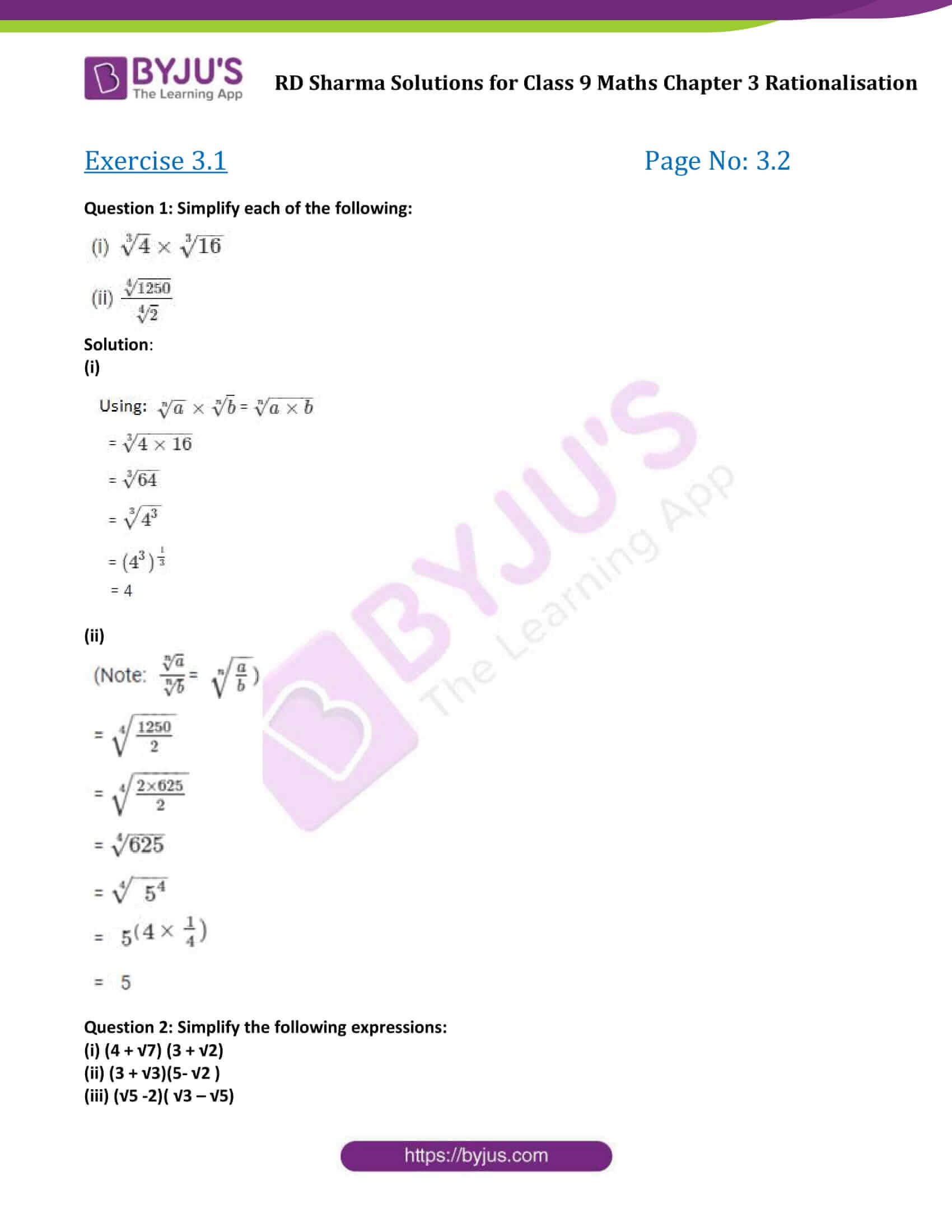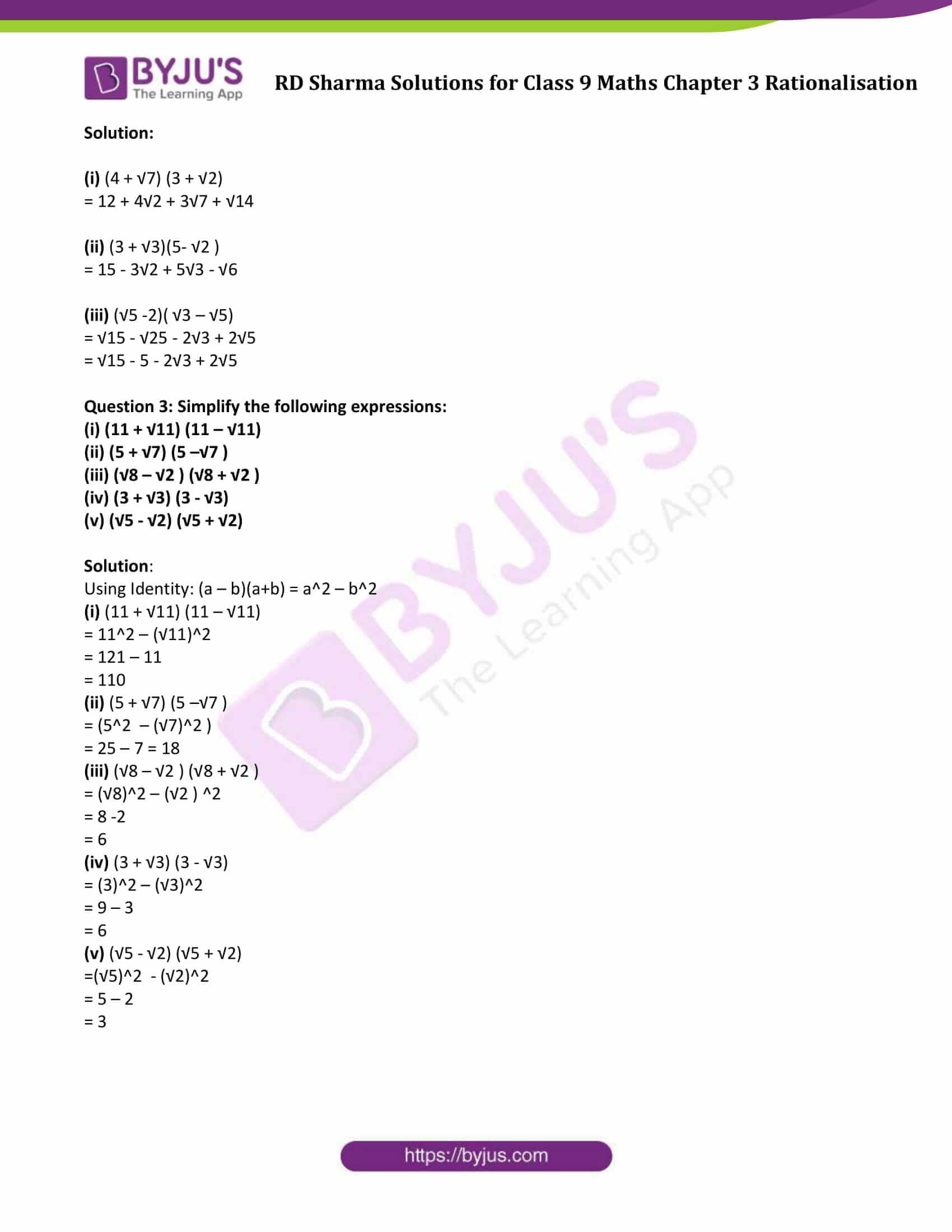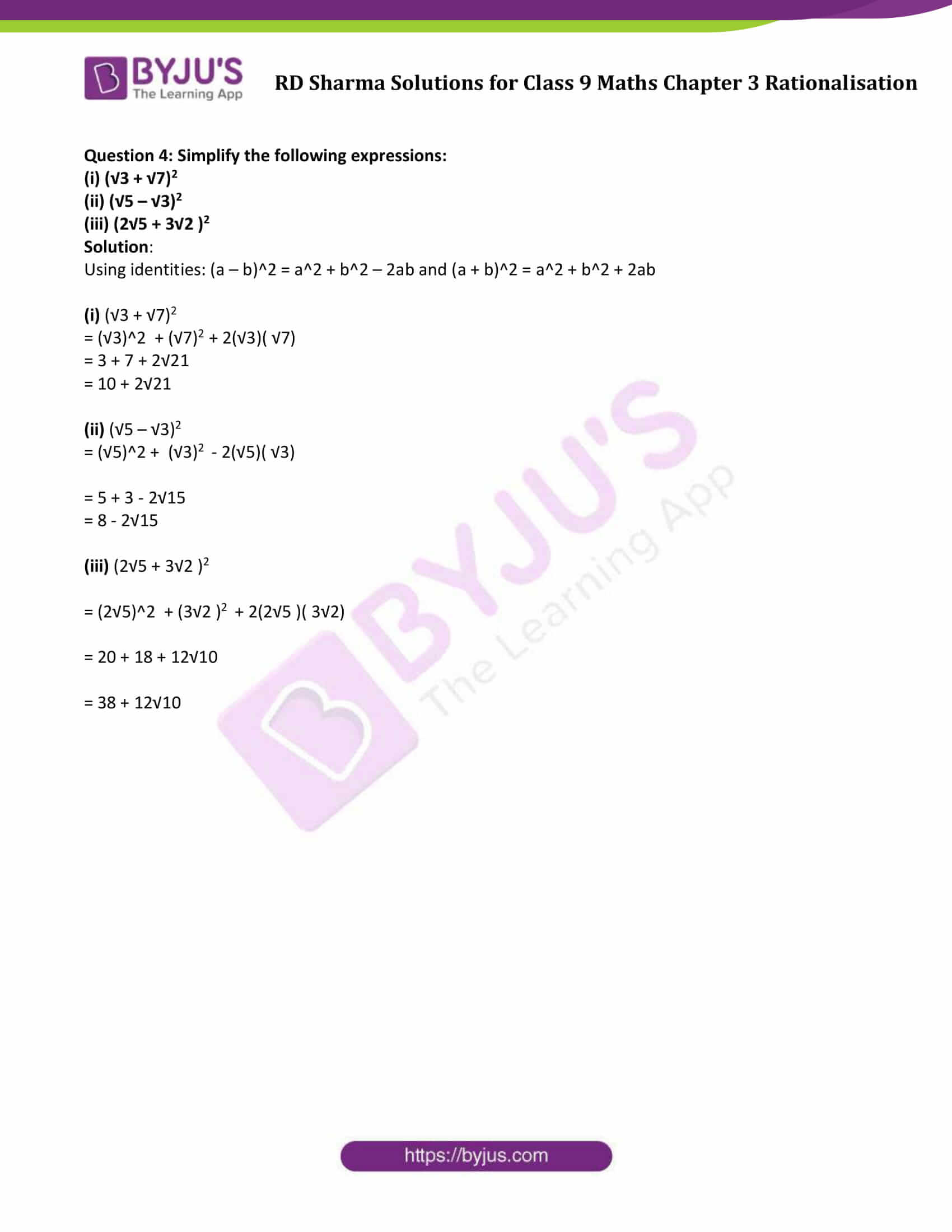### Access Answers to Maths RD Sharma Solutions for Class 9 Chapter 3 Rationalisation Exercise 3.1

Question 1: Simplify each of the following:Solution:

(i)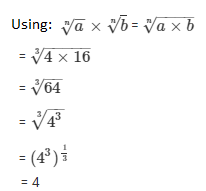(ii)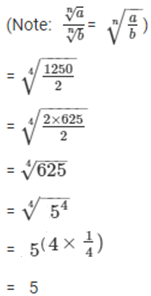Question 2: Simplify the following expressions:

(i) (4 + √7) (3 + √2)

(ii) (3 + √3)(5- √2 )

(iii) (√5 -2)( √3 – √5)

Solution:

(i) (4 + √7) (3 + √2)

= 12 + 4√2 + 3√7 + √14

(ii) (3 + √3)(5- √2 )

= 15 – 3√2 + 5√3 – √6

(iii) (√5 -2)( √3 – √5)

= √15 – √25 – 2√3 + 2√5

= √15 – 5 – 2√3 + 2√5

Question 3: Simplify the following expressions:

(i) (11 + √11) (11 – √11)

(ii) (5 + √7) (5 –√7 )

(iii) (√8 – √2 ) (√8 + √2 )

(iv) (3 + √3) (3 – √3)

(v) (√5 – √2) (√5 + √2)

Solution:

Using Identity: (a – b)(a+b) = a2 – b2

(i) (11 + √11) (11 – √11)

= 112 – (√11)2

= 121 – 11

= 110

(ii) (5 + √7) (5 –√7 )

= (52 – (√7)2 )

= 25 – 7 = 18

(iii) (√8 – √2 ) (√8 + √2 )

= (√8)2 – (√2 ) 2

= 8 -2

= 6

(iv) (3 + √3) (3 – √3)

= (3)2 – (√3)2

= 9 – 3

= 6

(v) (√5 – √2) (√5 + √2)

=(√5)2 – (√2)2

= 5 – 2

= 3

Question 4: Simplify the following expressions:

(i) (√3 + √7)2

(ii) (√5 – √3)2

(iii) (2√5 + 3√2 )2

Solution:

Using identities: (a – b)2 = a2 + b2 – 2ab and (a + b)2 = a2 + b2 + 2ab

(i) (√3 + √7)2

= (√3)2 + (√7)2 + 2(√3)( √7)

= 3 + 7 + 2√21

= 10 + 2√21

(ii) (√5 – √3)2

= (√5)2 + (√3)2 – 2(√5)( √3)

= 5 + 3 – 2√15

= 8 – 2√15

(iii) (2√5 + 3√2 )2

= (2√5)2 + (3√2 )2 + 2(2√5 )( 3√2)

= 20 + 18 + 12√10

= 38 + 12√10

## RD Sharma Solutions for Class 9 Maths Chapter 3 Rationalisation Exercise 3.1

RD Sharma Solutions Class 9 Maths Chapter 3 Rationalisation Exercise 3.1 is based on Some important Identities as listed below:

• Identity I: (a + b)2 = a2 + 2ab + b2
• Identity II: (a – b)2 = a2 – 2ab + b2
• Identity III: a2 – b2 = (a + b)(a – b)
• Identity IV: (x + a)(x + b) = x2 + (a + b)x + ab